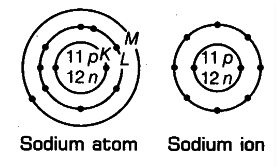# NCERT Exemplar Solutions Class 9 Science Solutions for Structure of the Atom - Exercise in Chapter 4 - Structure of the Atom

Show diagrammatically the electron distributions in a sodium atom and a sodium ion and also give their atomic number.

Atomic number of sodium (Z) =11 Mass number of sodium (A) = 23

Number of protons in the nucleus = 11 Number of neutrons in the nucleus =23-11 = 12

Number of electrons = 11

Electronic configuration of Na-atom = 2, 8, 1(K,L,M)

Na+ ion is formed from sodium atom by loss of an electron (present in the outermost shell). Hence,

the electronic configuration is 2, 8(K, L). However, the number of protons and neutrons remains the same.Related Questions
Exercises

Lido

Courses

Teachers

Book a Demo with us

Syllabus

Maths
CBSE
Maths
ICSE
Science
CBSE

Science
ICSE
English
CBSE
English
ICSE
Coding

Terms & Policies

Selina Question Bank

Maths
Physics
Biology

Allied Question Bank

Chemistry
Connect with us on social media!